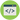# ABCs of Regular ExpressionThere are lot of programmer in world use RegEx just because his/her boss or friend or anybody ask him to use. But we should know why it is good to know RegEx. Let's say we need to check phone number of people like 444–235–1234. So here, first 3 digit may be code and then there is - then again 3 digit and again- and so on. User may enter 10 digit phone number directly without any hyphen.

So there is one option to check this, IF CONDTION. Write conditions in OR expression and get result. But it is complex to write and read it. There is also chance to get bug in this condition. So here we can use RegEx.

# How to use RegEx

Note that, in every language there is different way to use this. I'm going to show you in javascript ✨

• Every expression in Regex start with forward slash (/) and end with forward slash For example, I want match my name then
``````var myName = 'sanket';
myName.match(/regular expression/);``````
• We can place OR | symbol in between RegEx to match the string. For e.g `/san|sanket|ket/`
• If we want to match alphabets then we can write RegEx as `/[a-z]/`
• Note that in above RegEx, we are grouping a to z characters and it will match only one character. To match one more character we have to use + operator (i.e. Quantifiers). For e.g, we want to match character say `ar`, `arr`, `arrr` etc. Then we can write RegEx as `/ar+/` This will match single `a` with one or more `r`.
• So above RegEx will be `/[a-z]+/`. This RegEx will match any small-case character between a to z. What if we want to match between A to Z. Then we can also add another group like `/[a-zA-Z]+/`. This can match any alphabet between a to z OR A to Z.
• There is one more way to write above RegEx, `/[a-z]+/i` i is modifier, that means case insensitive which will match upper case and lower case.
• Now if we want to match whitespace then aboe RegEx will return false. So to do that we can use `/s` which includes white space, new line, tab. so our RegEx will be, `/[a-z/s]+/i` OR `/[/sa-z]+/i`. Now of course to use digit RegEx wll be `/[a-z/s0-9]+/i`
• We can refactor our character set, we can use `\w` is same as `[a-zA-Z0-9]`. So above RegEx will be `/[\w/s]+/`.

Cool, Right!

# Email Validation

• Lets say we have to match email address then our character set will be `/\[email protected]\w/`. But this going match only 1 word-like character. So we need to add `+`, `/\[email protected]\w/`
• Above RegEx will match only [email protected] email address [email protected]
• So to match `.` (dot) then RegEx will be, `/\[email protected]\w+./` and again for 'com' RegEx will be, `/\[email protected]\w+.\w+/`
• But above RegEx will also match `!` instead of dot so, we need to escape dot so, `/\[email protected]\w+\.\w+/` Not that `.` in RegEx means this is wildcard which matches any character except newline But in above regex there is one problem email id can be com | net | edu | org. We can't put any other word instead of above one so our regex will be, `/\[email protected]\w+\.(com|org|net|edu)/i`
• We need to make sure that there should be nothing garbage between start and end of email address. so to do that we have another symbol `^` : start looking at beginning of string/email `\$` : stop looking at end of string/email
• So our final Email RegEx will be, `/^\[email protected]\w+.(com|org|net|edu)\$/i`

# Other

• `?` is use to make character set optional and `\b` is use to match whole word. For e.g. We want to match ok, okay, okiee. Then regex will be `/\bok(ay)?\b/i`
• `^` means 'not' when place in character set
• `\d` means 'any number', so `/[^\d]+/i` means 'anything that's not number'
• `[^\d]` is same as `\D` : match every character except number (This is shorthand operator)
• `[^\s]` is same as `\S` : match every character except whitespace (This is shorthand operator)
• Lets say if we want to match just specific pattern to 2 time then regex will be `\[a-z]{2}\`. So regex will match any character b/w a-z exactly 2 times.we can specify range like, `[a-z]{1,3}` this will match the character in string a minimum of 1 time or maximum 3 time

# E-mail regular expression

Following regular expressions that validate e-mail addresses in order to find the best one. It matches most of email address

``/^(?!(?:(?:\x22?\x5C[\x00-\x7E]\x22?)|(?:\x22?[^\x5C\x22]\x22?)){255,})(?!(?:(?:\x22?\x5C[\x00-\x7E]\x22?)|(?:\x22?[^\x5C\x22]\x22?)){65,}@)(?:(?:[\x21\x23-\x27\x2A\x2B\x2D\x2F-\x39\x3D\x3F\x5E-\x7E]+)|(?:\x22(?:[\x01-\x08\x0B\x0C\x0E-\x1F\x21\x23-\x5B\x5D-\x7F]|(?:\x5C[\x00-\x7F]))*\x22))(?:\.(?:(?:[\x21\x23-\x27\x2A\x2B\x2D\x2F-\x39\x3D\x3F\x5E-\x7E]+)|(?:\x22(?:[\x01-\x08\x0B\x0C\x0E-\x1F\x21\x23-\x5B\x5D-\x7F]|(?:\x5C[\x00-\x7F]))*\x22)))*@(?:(?:(?!.*[^.]{64,})(?:(?:(?:xn--)?[a-z0-9]+(?:-[a-z0-9]+)*\.){1,126}){1,}(?:(?:[a-z][a-z0-9]*)|(?:(?:xn--)[a-z0-9]+))(?:-[a-z0-9]+)*)|(?:\[(?:(?:IPv6:(?:(?:[a-f0-9]{1,4}(?::[a-f0-9]{1,4}){7})|(?:(?!(?:.*[a-f0-9][:\]]){7,})(?:[a-f0-9]{1,4}(?::[a-f0-9]{1,4}){0,5})?::(?:[a-f0-9]{1,4}(?::[a-f0-9]{1,4}){0,5})?)))|(?:(?:IPv6:(?:(?:[a-f0-9]{1,4}(?::[a-f0-9]{1,4}){5}:)|(?:(?!(?:.*[a-f0-9]:){5,})(?:[a-f0-9]{1,4}(?::[a-f0-9]{1,4}){0,3})?::(?:[a-f0-9]{1,4}(?::[a-f0-9]{1,4}){0,3}:)?)))?(?:(?:25[0-5])|(?:2[0-4][0-9])|(?:1[0-9]{2})|(?:[1-9]?[0-9]))(?:\.(?:(?:25[0-5])|(?:2[0-4][0-9])|(?:1[0-9]{2})|(?:[1-9]?[0-9]))){3}))\]))\$/iD``

For Javascript -

``/^[-a-z0-9~!\$%^&*_=+}{\'?]+(\.[-a-z0-9~!\$%^&*_=+}{\'?]+)*@([a-z0-9_][-a-z0-9_]*(\.[-a-z0-9_]+)*\.(aero|arpa|biz|com|coop|edu|gov|info|int|mil|museum|name|net|org|pro|travel|mobi|[a-z][a-z])|([0-9]{1,3}\.[0-9]{1,3}\.[0-9]{1,3}\.[0-9]{1,3}))(:[0-9]{1,5})?\$/i;``

Test Cases are Here

Happy Coding 🔥